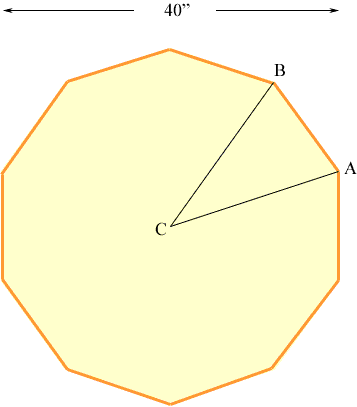Quandaries and Queries I need to fabricate a 10 section, 4" pipe circle with an inside diameter of 40". I would like to know what angles would apply and how to find them, thank you Gil Hi Gil, I drew a diagram of what I think you are describing.AB is one of the 10 lengths of pipe and C is the center of the region. Since there are 10 lengths of pipe the angle BAC is 360/10 = 36o. The sum of the angles in a triangle is 180o and hence angle ABC is (180 - 36)/2 = 72o. Thus the angle at the end of each length of pipe is 72o. Penny Go to Math Central